Geometry

# Triangles - Classification by Sides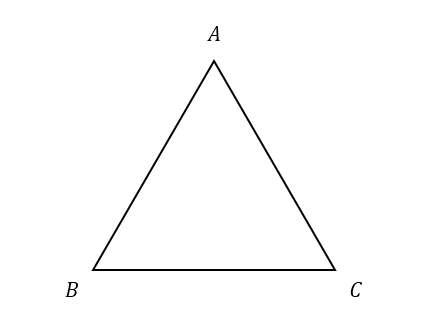If the above triangle is an equilateral triangle and the length of $$\overline{AB}$$ is $$\lvert \overline {AB} \rvert =11 ,$$ what is $$\lvert \overline {AC} \rvert ?$$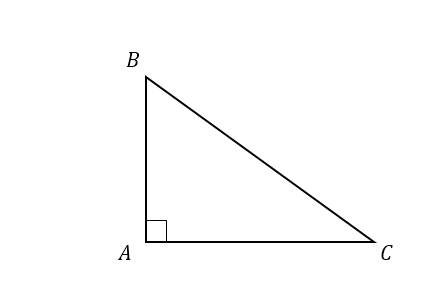If $$\triangle ABC$$ is a right triangle and the lengths of two sides are $$\lvert \overline {AB} \rvert = 6$$ and $$\lvert \overline {AC} \rvert = 8 ,$$ what is $$\lvert \overline {BC} \rvert ?$$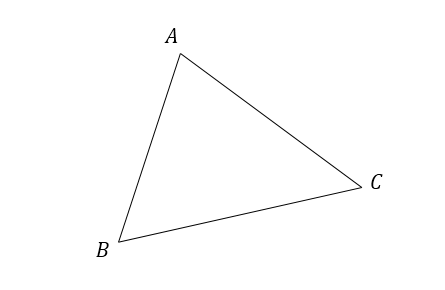$$\triangle {ABC}$$ is an acute triangle with side lengths $$\lvert \overline {AB} \rvert = 120$$ and $$\lvert \overline {AC} \rvert = 160.$$ If $$\lvert \overline {BC} \rvert \geq \lvert \overline {AC} \rvert,$$ what is the possible range of $$\lvert \overline {BC}\rvert?$$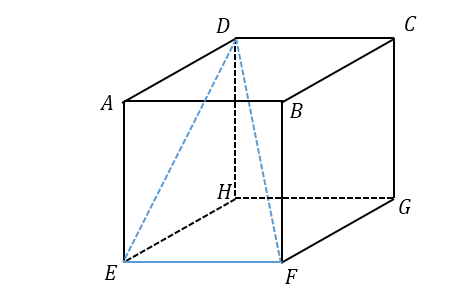Solid figure $$ABCDEFGH$$ is a regular cube with side length $$12.$$ What is the area of $$\triangle DEF ?$$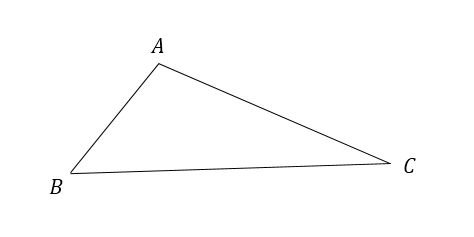Angle $$\angle A$$ is an obtuse angle in triangle $$ABC$$ with side lengths $$\lvert \overline {AB} \rvert = 30$$ and $$\lvert \overline {AC} \rvert = 40.$$ If $$\lvert \overline {BC} \rvert$$ is an integer and $$\lvert \overline {BC} \rvert \geq \lvert \overline {AC} \rvert,$$ what is the minimum value of $$\lvert \overline {BC}\rvert ?$$

×